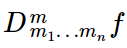# Mixed Derivative (Partial, Iterated)

The mixed derivative (also called a mixed partial derivative) is a second order derivative of a function of two or more variables. “Mixed” refers to whether the second derivative itself has two or more variables. For example:

• fxy and fyx are mixed,
• fxx and fyy are not mixed.

## Mixed Derivative Example

The function of two variables f(x, y) can be differentiated with respect to x or y, giving two first order partial derivatives ∂f / ∂x and ∂f / ∂y. These can be differentiated again with respect to x and y, giving rise to four different second order derivatives:

A and B are called “unmixed derivatives” because they contain the same variables (xx, yy); C and D are called “mixed derivatives”. Together, all four are iterated partial derivatives of second order.

The variable which appears first is generally the one you would want to differentiate with respect to first. For example, fyx means that you should differentiate with respect to y, then differentiate with respect to x. However, if all the derivatives within the mixed derivatives are continuous, you can make a series of interchanges between x and y. For example, you could write them so that differentiations to x all come first, as in fxyy or fxxy (Brand, 2013).

Example question: Find the mixed derivatives of f(x, y) = x2 y3.

Solution Steps:
Step 1: Find the first partial derivatives.

• With respect to x (holding y constant): fx = 2xy3
• With respect to y (holding x constant): fy = 3x22

Note: The term “hold constant” means to leave that particular expression unchanged. In this example, “hold x constant” means to leave x2 “as is.” Likewise, hold y constant means that you leave y3 as is.

Step 2: Find the second order derivatives. There are four:

• fxx = 2y3
• fxy = 6xy2
• fyx = 6xy2
• fxx = 6x2y

Step 3: Identify the mixed partial derivatives. There are two:

• fxx = 2y3
• fxy = 6xy2
• fyx = 6xy2
• fxx = 6x2y

## Notation for Mixed Derivative

The curly d (∂) is usually used as notation for a mixed derivative. Other notations you might see (especially in older texts) include D2xyz and zxy. For higher-order derivatives, fxy′ is often used (Berry et al., 1989).

## Note of Number of Indices

Technically, a mixed derivative refers to any partial derivativewith two or more non-zero indices mi. For example f(x, y, z) or f(g, h, k). However, functions of two variables are more common. In addition, listing mixed derivatives for functions of more than two variables can quickly become quite confusing to keep track of all the parts. While a function of two variables has four mixed partial derivatives, a function of three variables has 27 ! (xxx, xxy, xxz, xyy, xyz…).

## References

Berry, J. et al. (1989). Introductory Mathematics Through Science Applications. Cambridge University Press.
Brand, L. (2013). Advanced Calculus: An Introduction to Classical Analysis. Dover Publications.
Newcastle University (2018). Partial Derivatives. Retrieved August 28, 2020 from: https://internal.ncl.ac.uk/ask/numeracy-maths-statistics/core-mathematics/calculus/partial-derivatives.html#:~:text=Second%20Order%20and%20Mixed%20Derivatives&text=The%20second%20order%20derivatives%20f,to%20more%20than%20one%20variable.
Tesler, P. (2018). Iterated Partial Derivatives. Retrieved August 28, 2020 from: https://www.math.ucsd.edu/~gptesler/20c/slides/20c_itderivs_f18-handout.pdf

CITE THIS AS:
Stephanie Glen. "Mixed Derivative (Partial, Iterated)" From StatisticsHowTo.com: Elementary Statistics for the rest of us! https://www.statisticshowto.com/mixed-derivative-partial-iterated/